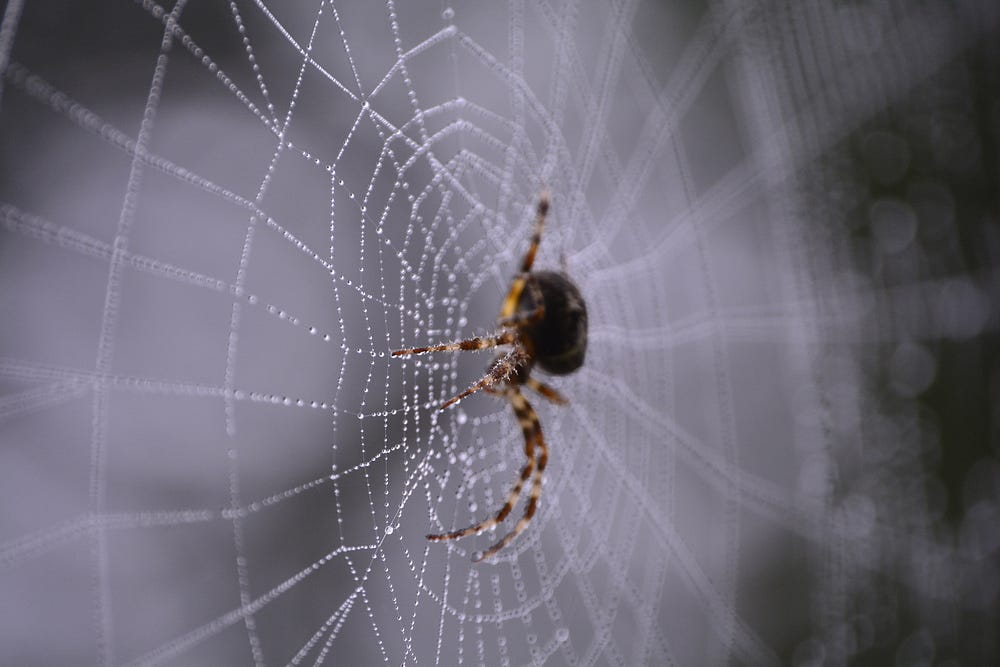# Image Super-Resolution using Convolution Neural Networks and Auto-encoders

Original article was published on Deep Learning on MediumModel building

Lets define the structure of model. Moreover, to overcome the possibility of over-fitting, we are using l1 regularization technique in our convolution layer.

`Input_img = Input(shape=(80, 80, 3)) #encoding architecturex1 = Conv2D(64, (3, 3), activation='relu', padding='same', kernel_regularizer=regularizers.l1(10e-10))(Input_img)x2 = Conv2D(64, (3, 3), activation='relu', padding='same', kernel_regularizer=regularizers.l1(10e-10))(x1)x3 = MaxPool2D(padding='same')(x2)x4 = Conv2D(128, (3, 3), activation='relu', padding='same', kernel_regularizer=regularizers.l1(10e-10))(x3)x5 = Conv2D(128, (3, 3), activation='relu', padding='same', kernel_regularizer=regularizers.l1(10e-10))(x4)x6 = MaxPool2D(padding='same')(x5)encoded = Conv2D(256, (3, 3), activation='relu', padding='same', kernel_regularizer=regularizers.l1(10e-10))(x6)#encoded = Conv2D(64, (3, 3), activation='relu', padding='same')(x2)# decoding architecturex7 = UpSampling2D()(encoded)x8 = Conv2D(128, (3, 3), activation='relu', padding='same', kernel_regularizer=regularizers.l1(10e-10))(x7)x9 = Conv2D(128, (3, 3), activation='relu', padding='same', kernel_regularizer=regularizers.l1(10e-10))(x8)x10 = Add()([x5, x9])x11 = UpSampling2D()(x10)x12 = Conv2D(64, (3, 3), activation='relu', padding='same', kernel_regularizer=regularizers.l1(10e-10))(x11)x13 = Conv2D(64, (3, 3), activation='relu', padding='same', kernel_regularizer=regularizers.l1(10e-10))(x12)x14 = Add()([x2, x13])# x3 = UpSampling2D((2, 2))(x3)# x2 = Conv2D(128, (3, 3), activation='relu', padding='same')(x3)# x1 = Conv2D(256, (3, 3), activation='relu', padding='same')(x2)decoded = Conv2D(3, (3, 3), padding='same',activation='relu', kernel_regularizer=regularizers.l1(10e-10))(x14)autoencoder = Model(Input_img, decoded)autoencoder.compile(optimizer='adam', loss='mse', metrics=['accuracy'])`

You can modify this model as per your choice and requirement to get better results. You can change number of layers, number of units or some regularization techniques too. For the time being, let’s move forward and see what our model looks like!

`autoencoder.summary()`